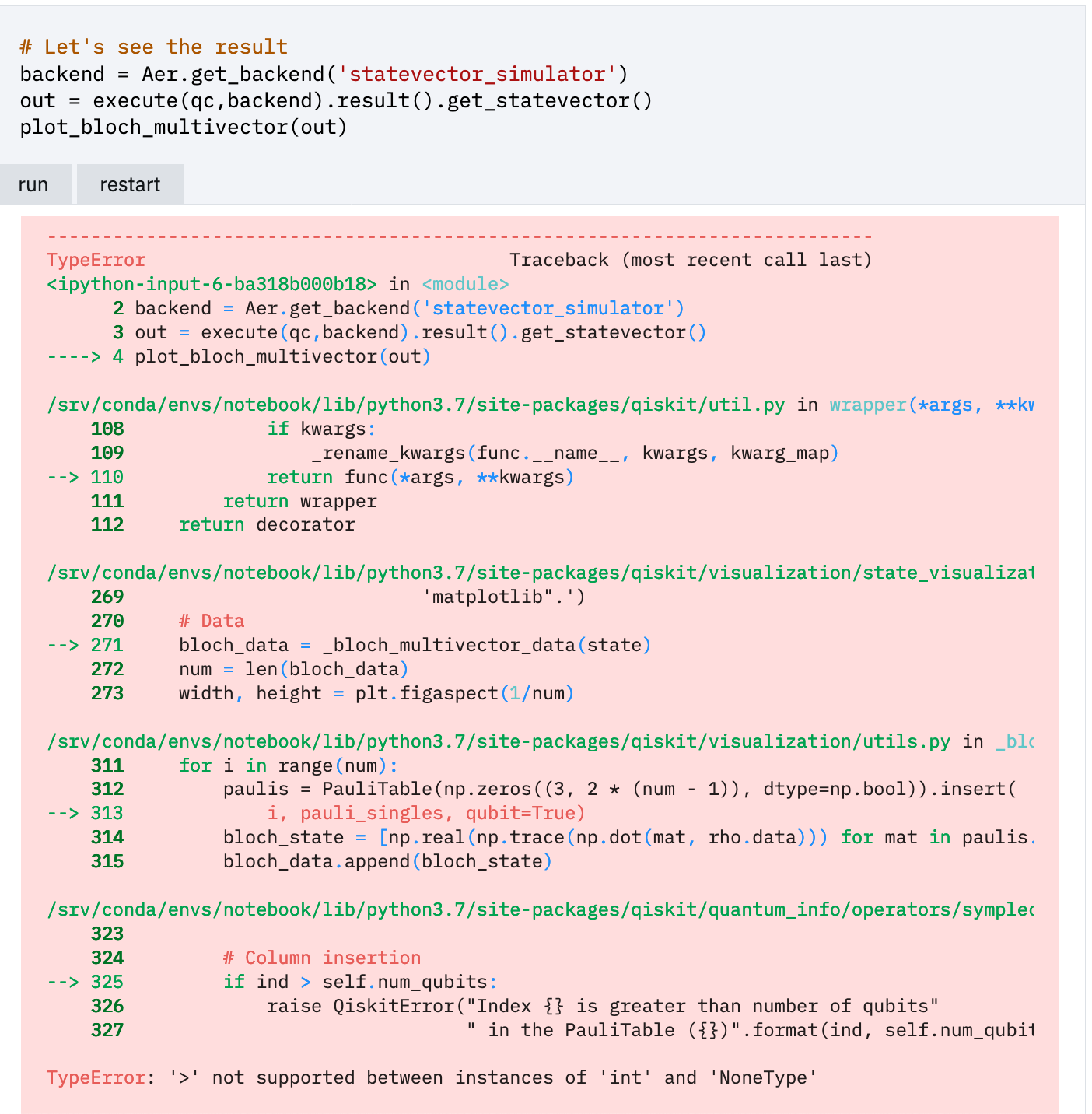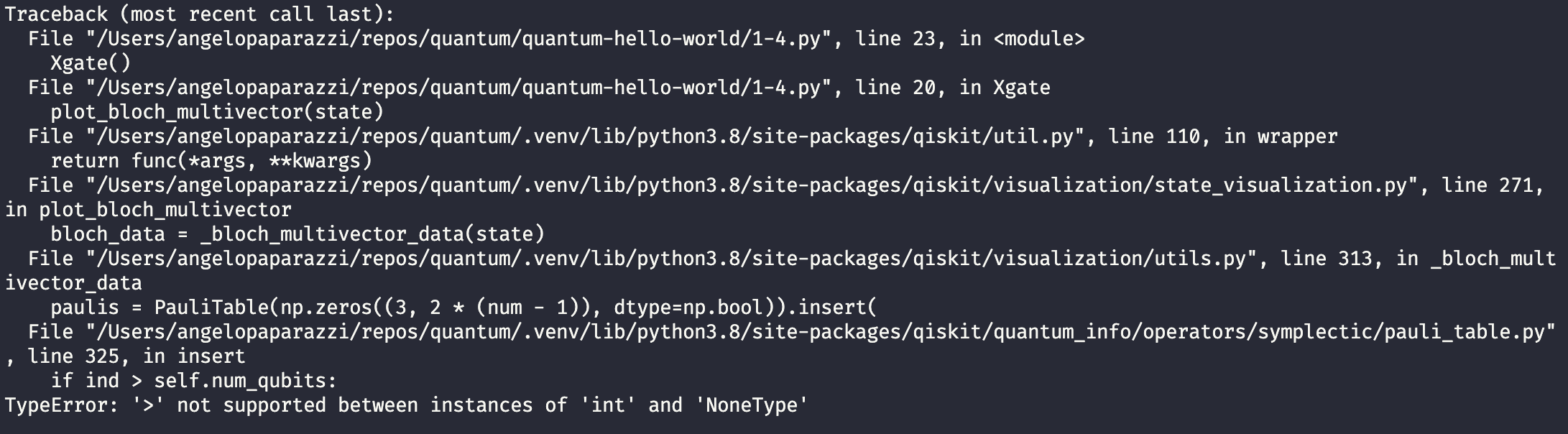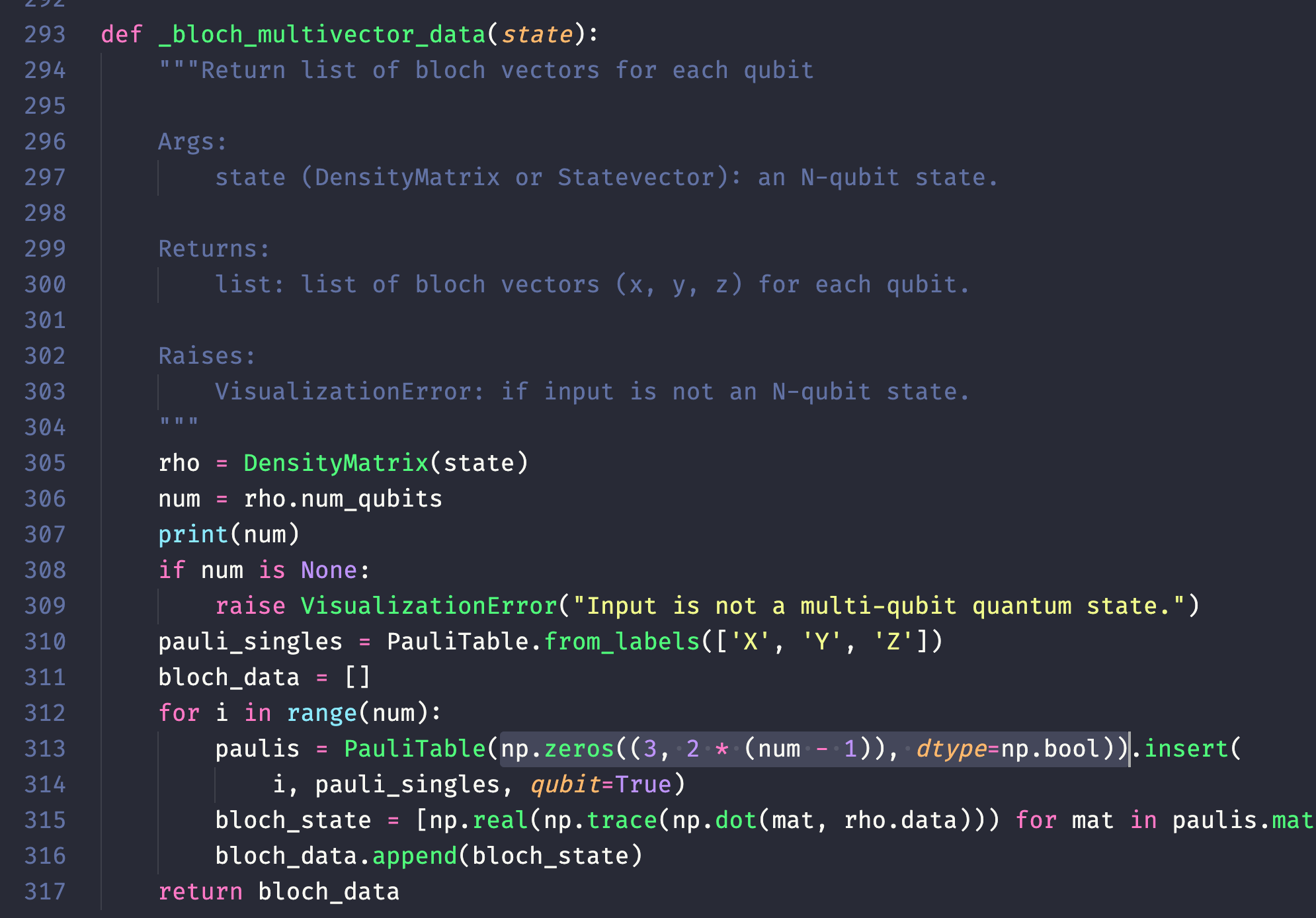# plot_bloch_multivector does not accept single qubit states and is used in the Qiskit Textbook

### Informations

• Qiskit version: {'qiskit-terra': '0.15.1', 'qiskit-aer': '0.6.1', 'qiskit-ignis': '0.4.0', 'qiskit-ibmq-provider': '0.8.0', 'qiskit-aqua': '0.7.5', 'qiskit': '0.20.0'}
• Python version: 3.8.5
• Operating system: 10.15.5 (Catalina)

To start, I was unsure whether to report this as a bug or an issue in the Qiskit Textbook but I will post the issue and potential solution.

### What is the current behavior?

The `plot_bloch_multivector` function imported from `qiskit.visualization` throws a TypeError when passed a single qubit state: `TypeError: '>' not supported between instances of 'int' and 'NoneType'`

### Why this is an issue in Qiskit Textbook as well

Section 1.4: Single Qubit Gates Subsection 1.1: The X-Gate There is a code snippet here that falls prey to using a single qubit state and throws the error described above when run### Steps to reproduce the problem

``````
from qiskit import QuantumCircuit
from qiskit.quantum_info import Statevector
from qiskit.visualization import plot_bloch_multivector

qc = QuantumCircuit(1)
state = Statevector.from_instruction(qc)
plot_bloch_multivector(state)
``````### What is the expected behavior?

If `plot_bloch_multivector` is only supposed to handle multi qubit states (as I would guess by the name) then a more graceful and informational error would be expected. If this is not the case, then the function must be fixed to plot single qubit states.

### Suggested solutions

I would look to this function found in `.venv/lib/python3.8/site-packages/qiskit/visualization/utils.py`It appears on line 308 that the number of qubits is checked in case it is not a multi-qubit quantum state; however, instead of checking for `num < 2 || num is None` it only checks if num is None. This then creates the root of the issue on line 313 where the function `np.zeros((3, 2 * (num - 1)), dtype=np.bool))` creates an empty array.

• 点赞
• 写回答
• 关注问题
• 收藏
• 复制链接分享
• 邀请回答

#### 5条回答

• No worries , good luck!

点赞 评论 复制链接分享
• Hi , thanks for reporting. This looks like a duplicate of https://github.com/Qiskit/qiskit-terra/issues/4941 which was fixed by PR https://github.com/Qiskit/qiskit-terra/pull/4948 (and will be included in the next qiskit terra release). Feel free to re-open if not.

点赞 评论 复制链接分享
• Yep looks like the issue was already resolved👍🏼

点赞 评论 复制链接分享
• Is it alright if I try fixing this issue?

点赞 评论 复制链接分享
• Hi -ebbs thanks for asking. I was actually planning to start contributing to the Qiskit project as part of my journey to learn quantum computing so I would like to tackle this myself. I would just like clarification to know if the intention of `plot_bloch_multivector` is to only plot multi-qubit quantum states or if single qubit states should also be plotted.

点赞 评论 复制链接分享